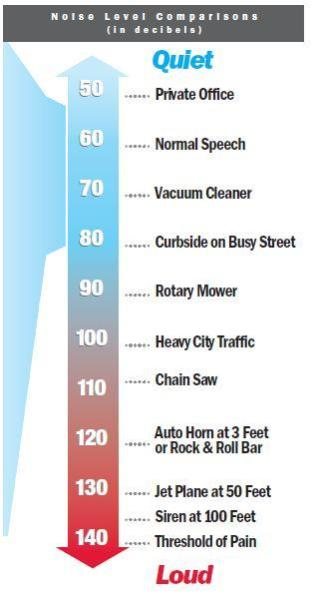Call Toll Free: 1-800-882-4959
 Common terms used when discussing generators: Alternating Current:  An electric current that reverses direction at regular intervals, having a magnitude that varies continuously in sinusoidal manner.  Abbreviation - A/C  See Also - Direct Current. Amperage:  The strength of an electric current measured in amperes.  Abbreviation - amp.  Amps = watts/volts Amplitude:  The amount by which a voltage or current changes from zero or an average value.  The maximum deviation of an alternating current from its average value. Apparent Power:  A normal multimeter can measure volts, electrical pressure, and amps, electrical current.  The product of these two measurements is apparent power, because it is the apparent power transfer.  Apparent power is measured in volt-amps (or Kilovolt-amps KVA), because it is the product of volts (root-mean-squared) and amps (also root-mean-squared).  The problem with a multimeter is that it averages these quantities over time.Decibels:  A unit used to express the intensity of a sound wave.  Abbreviation - dB.  See chart on right for common dB levels Direct Current:  An electric current of constant direction, having a magnitude that does not vary or varies only slightly.  Abbreviation - D/C  See Also - Alternating Current Frequency:  The rate at which a repeating event occurs, such as the full cycle of a wave.  Frequencies are usually measured in hertz.  Abbreviation - freq.  See Also - Hertz, Amplitude Hertz:  The international unit of frequency:  One cycle per second.  Abbreviation - Hz.  Household current in the United States is 60 Hz Kilowatts (kW):  A unit of power equal to 1000 watts. (Real Power)  Abbreviation - kW.  See also Watts Kilovoltampere (KVA):  A volt-ampere in electrical terms is the amount of power in an alternating current circuit equal to a current flow of one ampere at an electrical force of ove volt.  It is equivalent to watts for non-reactive circuits and in the strictest sense is identical, but by convention the two are used as units for subtly different physical quantities.  While the KVA and the Kw are dimensionally equivalent on may find products rated in both with different numbers.  This is common practice on uninterruptible power supplies (UPS).  The KVA rating is the apparent power that a UPS is capable of producing, while the Kw rating is the real power it is capable of producing.  KVA divided by the Kw is referred to as the power factor. Load:  The power delivered by a generator.  Also the devise that receives the power.  When you power a device with a generator you place a load on the generator, the device being powered is the load. Power Factor:  The ratio of real power (kW) flowing to the load to the apparent power, and is a number between 0 and 1 frequently expressed as a percentage.  Due to energy stored in the load and returned to the source, or due to a non-linear load that distorts the wave shape of the current drawn from the source, the apparent power can be greater than the real power.  In an electric power system, a load with low power factor draws more current than a load with a high power factor for the same amount of useful power transferred.  The higher currents increase the energy lost in the distribution system, and require larger wires and other equipment. Real Power:  Real power is measured in watts (also Kilowatts kW).  As the waves of current and voltage coincide less, less real power is transferred. Sinusoid (Sine Wave):  Graphing the voltage of an alternating current gives a sine wave pattern. Standby:  A generator that provides power when conventional power is interrupted. Volt:  The international system unit of electric potential and electrovotive force, equal to the difference of electric potential between two points on a conducting wire carrying a constant current of one ampere when the power dissipated between the points is one watt. Watt:  In electricity a watt is equal to current (in amperes) multiplied by voltage (in volts).
 Generators Honda Gas Generators Multiquip Gas Generators Generator Basics Generator Definitions Diesel Generators Generator / WeldersMQ Generator Handbook